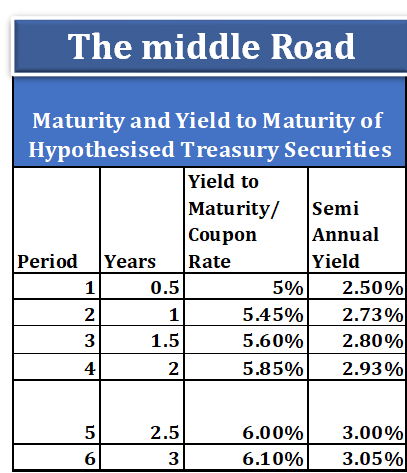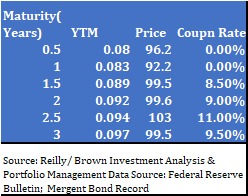Nishant Malhotra Founder of Middle Road OPC Pvt Ltd & The middle Road platform talks about the startup

### Ahoy Ahoy-Ahoy!

Welcome to the Kickass platform enabling social change & impact

# Valuation Module 3 : Term Structure of Interest Rates Problems and Solutions 1

You are here:

The following are set of questions with solutions related to the module on Term Sheet of Interest Rates1. Look at the spot rates given for different periods in the fig(left) . Consider a time period one one year. Calculate one year forward rates  for the data given below. Refer to the excel attached in Module 3 on valuation for the workings and answers.

The excel sheet includes the discount rates and embedded formulas to calculate the forward rate.

 (1+fp,q)^q-p = (1+Sq)^q/(1+Sp)^p               q>p where they depict time periods

2.  Consider a 7% coupon rate bond, 4 year semi annual coupon payment note. The par value of the bond is 100. The spot rates of the time periods are as follows. The rates for the following time periods ( 1 to 8) respectively are as follows: 5%, 5.25%, 5.60%, 5.95%, 6.10%, 6.30%, 6.39%, 6.55%.  Using these spot rates, calculate the price of the bond.  Ans 101.783. Refer to the question on YTM coupon rates sheets on the term rates excel sheet attached in Valuation Module 3 : Term Structure of Interest Rates. You can consider par vale as 100 or 1000.The maturity and yield to maturity of the hypothesized treasury securities are mentioned. There are six periods of six months each. The periods range from 6 months to 3 years. Use this information to arrive at all the spot rates using the technique of bootstrapping. The six months and one year are zero-coupon bonds with 5% and 5.45% annualized yields. Calculate spot rates for all the periods.

Refer to the excel sheet Question YTM Coupon Rates for the solution. Side, calculation of spot rate for the fourth year is explained. In this question, a par value of 1000 is used although you can use 100, you will arrive at the same answer. For cash flows, refer to the YTM for the fourth period. All cash flows should be discounted at respective spot rates. Remember for the tutorial, yields of zero-coupon bonds are spot rates.

Use the present value method to derive the spot rates. The present value in the fourth year is 1000- 83.19 = 916.81. The present value of the cash flows is discounted to the par value. Herein, the par value is 1000.

4. List true or false questions related to Term Structure of Interest Rates

a. A bond has a semi annual rate of 2.8%, the annualized rate is 5.6%

b. Yields at the shorter end of the curve are 0.25% while the 30 year yield is at 1.5%. This is a steepener.

c. While calculating the yield to maturity of the bond, one of the assumption is that the coupons are reinvested at the computed YTM rate.

d. The inverse curve is a reasonable predictor  of future recessions in the US based on the Credit Suisse data since 1978.

e.  As the required yield on a bond increases, the price of the bond increases.

f. When the bond is trading at a discount, the current yield of the bond is greater than its YTM.

Ans ( True (2.8% * 2=5.6%, True The maturity spread between long term an short term is more than 300bps which is 0.03,  True, True, False( as the required yield increases, the present value of the cash flows i.e.. coupons and the maturity value reduces, reducing the price of the bond, False).

5.

Find the price of a 7 year Zero Coupon bond which has a required yield of 6.5% with a par value of 100.

Soln: For a zero coupon bond multiple the time period by 2 and divide the yield by 2.

P=M/(1+r) ^n where M= maturity value = 100  n= 7*2 = 14  r=3.25%

P =100/(1.0325)^14                   = 100/1.56         = 63.91

6.The following is a problem from the book by Reilly/ Brown. Based on the data, calculate the theoretical spot rate curve.  The price of the hypothetical treasury securities is given along with maturity and YTM is given.

Six month and one year treasury bills are zero coupon bonds have YTM given which is the annualized spot rate. Convert annualized into semi annualized,  use first to calculate spot rate for 1.5 years. Use spot rates to discount cash flows using coupon rate for 1.5 years. Equate the cash flows to the price of 1.5 years. Once you get the spot rate for 1.5 year go to 2 year. Use respective coupon rates and price to calculate all the spot rates.

Ans: Theoretical Spot rates from 0.5 to 3 years (0.08, 0.083, 0.08930, 0.09247, 0.09468, 0.09787) The rates are annualized